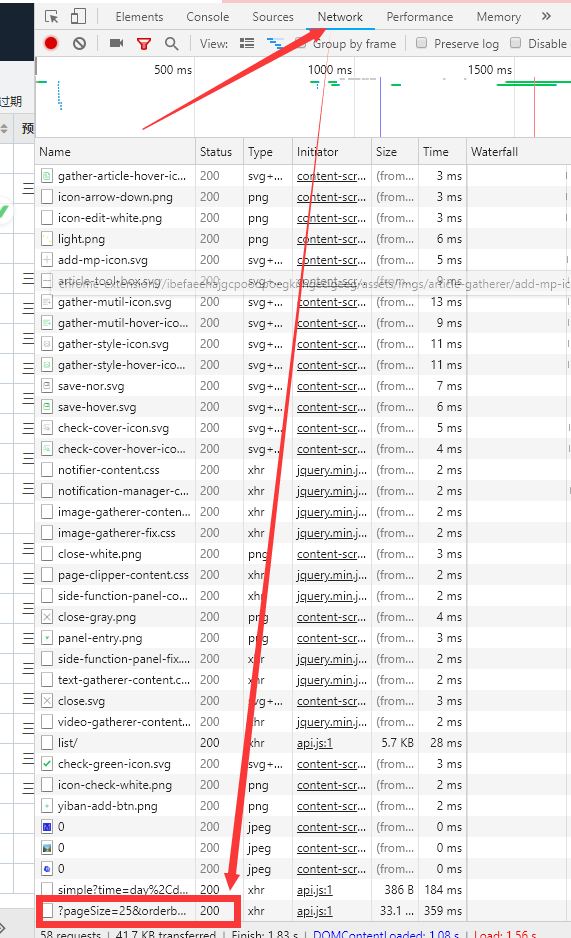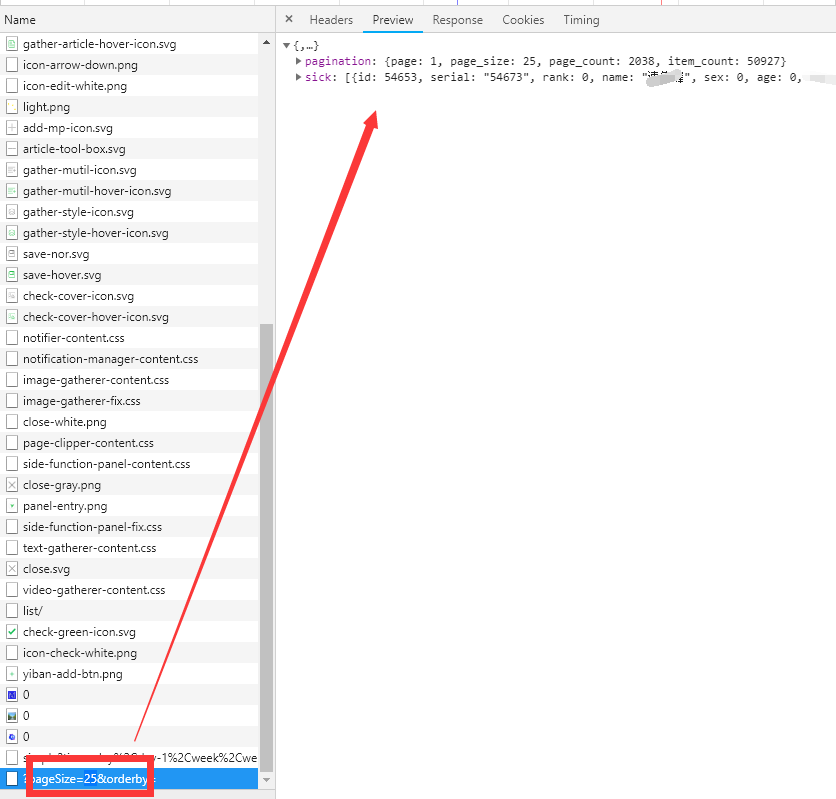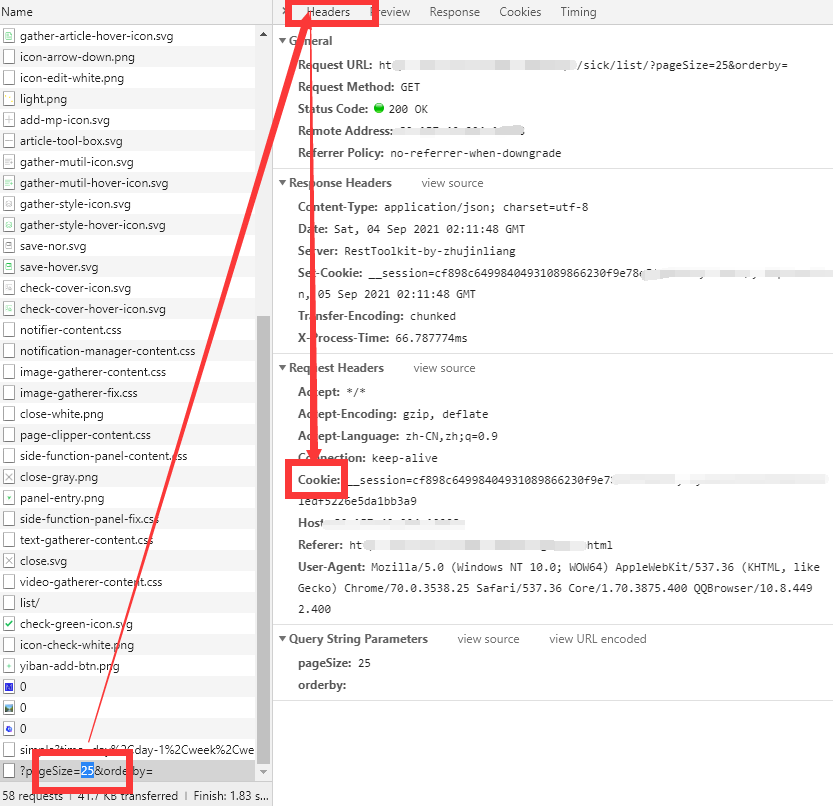//下面是允许请求跨域，跨域删除
/*作者：田小檬

*/

$headers = array( 'Authorization:'.'bearer 87d1a782-193b-423a-8097-fb8285f6bc05', );//授权认证$curl = curl_init(); // 启动一个CURL会话
curl_setopt($curl, CURLOPT_URL,$urls); // 要访问的地址
curl_setopt($curl, CURLOPT_SSL_VERIFYPEER, 0); // 对认证证书来源的检查 curl_setopt($curl, CURLOPT_SSL_VERIFYHOST, 0); // 从证书中检查SSL加密算法是否存在
curl_setopt($curl, CURLOPT_USERAGENT,$_SERVER['HTTP_USER_AGENT']); // 模拟用户使用的浏览器
curl_setopt($curl, CURLOPT_HTTPHEADER,$headers);

//添加POST请求如果需要请添加参数
/*作者：田小檬

*/
curl_setopt($curl, CURLOPT_POST,1); curl_setopt($curl, CURLOPT_POSTFIELDS, $post_data); // curl_setopt($curl, CURLOPT_FOLLOWLOCATION, 1); // 使用自动跳转
curl_setopt($curl, CURLOPT_AUTOREFERER, 1); // 自动设置Referer curl_setopt($curl, CURLOPT_COOKIE, $cookies); // 带上COOKIE请求 curl_setopt($curl, CURLOPT_TIMEOUT, 30); // 设置超时限制防止死循环
curl_setopt($curl, CURLOPT_HEADER, 0); // 显示返回的Header区域内容 curl_setopt($curl, CURLOPT_RETURNTRANSFER, 1); // 获取的信息以文件流的形式返回
$result = curl_exec($curl); // 执行操作
// $add = json_decode($result, true);//如果返回json请取消注释
/*作者：田小檬

*/
if (curl_errno($curl)) { echo 'Errno'.curl_error($curl);//捕抓异常
}
curl_close($curl); // 关闭CURL会话 return$result;//如果返回json请注释
*/